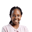Related Tags

julia
dataframes
communitycreator

# What are DataFrames in Julia?Joy Kareko

### Overview

A DataFrame is a 2-dimensional data structure that lets us store data in form of rows and columns, the way a spreadsheet does.

Loading our data into a data frame helps us view, manipulate, visualize and perform analytics on our data.

### DataFrames in Julia

In Julia, we have a DataFrame package dataframes.jl that lets you create/view the data from a CSV/Excel or any other type of file in tabular form with ease, for manipulation, analysis, and visualization of our data.

This package’s functionality is actually similar to that of Pythons’ pandas package.

To use the DataFrames package, we have to download and import it.

julia> ]
(v1.0) pkg>


To use it, we use the keyword using.

using DataFrames


For an example, let’s create a sample dataset for our use.

using DataFrames

name = ["Emma","David","Sara"]
salary =[100,300,600]
department = ["IT","Data","Data"]

df=DataFrame(;name,salary,department)



Notice that Julia uses double quotes " " when specifying string objects. This displays the data in tabular format.

The other use of the DataFrames package is loading data from a CSV file to a DataFrame.

df = DataFrame(load("mydata.csv"))


We will, however, have to use the DataFrames package in addition to Queryverse for that because of the load functionality that is available in Queryverse.

We will need to specify the two packages in our notebooks to use them:

using QueryVerse,DataFrames


In Julia, we’ll often find ourselves using the DataFrames package together with other existing packages, depending on what we would like to achieve with our data.

RELATED TAGS

julia
dataframes
communitycreator

CONTRIBUTORJoy Kareko
RELATED COURSES

View all Courses

Keep Exploring

Learn in-demand tech skills in half the time